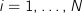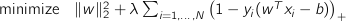# CVXGEN: Code Generation for Convex Optimization

## Example: Support vector machine (SVM)

This example, from machine learning, demonstrates the creation of a support vector machine (SVM). For some more details, see Boyd and Vandenberghe,8.6.1.

## Optimization problem

We are given observations

•, for, and a parameter

•We wish to choose two optimization variables: a weight vector

•, and offset

•to solve the optimization problem## CVXGEN code

dimensions
N = 50  # observations.
n = 10  # support vector dimension.
end

parameters
x[i] (n), i=1..N
y[i], i=1..N
lambda positive
end

variables
w (n)  # weight vector.
b  # offset.
end

minimize
quad(w) + lambda*sum[i=1..N](pos(1 - y[i]*(w'*x[i] - b)))
end